Like   Tweet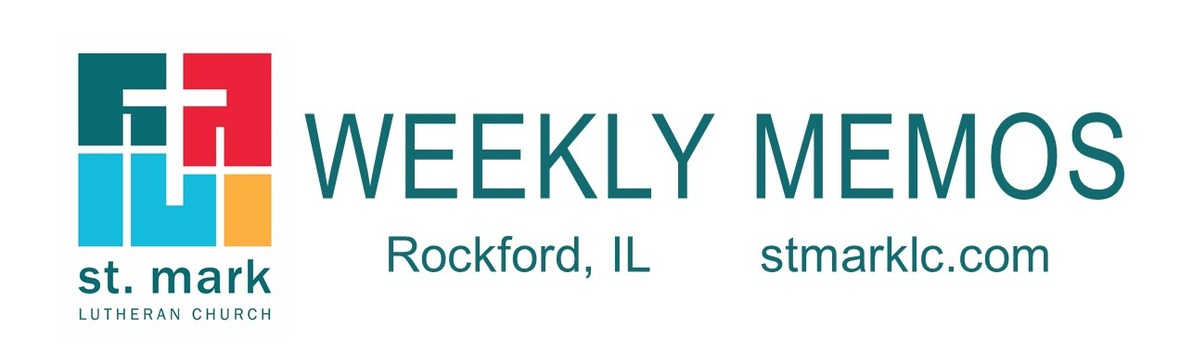table div table+table div table{width:100%;padding:0}table div table+table div table img{width:96.23%;padding:0;float:none}table div table+table div table td{width:100%;padding:0 1.88% 18px}/* styles */## Sunday, June 13 Confirmation Sunday

9:00 A.M. Indoor Worship Live-Stream in the Sanctuary
9:00 A.M. Confirmands will Affirm their Baptism (class of 2020)
10:00 A.M. Coffe and Donut Hour between services
11:00 A.M. Worship on the Lawn

## Monday, June 14

5:00 P.M. Children's Ministry
5:30 P.M. Rockford Ukulele Group

## Tuesday, June 15

10:00 A.M. Staff Meeting
11:30 A.M. St. Mark Duplicate Bridge - in the cafe

## Wednesday, June 16

6:30 P.M. Youth Group at St. Mark

## Thursday, June 17

9:00 A.M. Cherry Valley Bridge Group in Cafe
6:30 P.M. Blue Lights
6:30 P.M. Emotions Anonymous

## Friday & Saturday, June 18 & 19

Synod Assembly - Digital Event via Zoom

## Sunday, June 20 Father's Day

9:00 A.M. Indoor Worship Live-Stream in the Sanctuary
10:00 A.M. Coffee and Donut Hour between services
11:00 A.M. Worship on the Lawn

 table div table+table+table+table+table div table{width:100%;padding:0}table div table+table+table+table+table div table img{width:96.23%;padding:0;float:none}table div table+table+table+table+table div table td{width:100%;padding:0 1.88% 18px}/* styles */## News and Events

 table div table+table+table+table+table+table+table div table{width:100%;padding:0}table div table+table+table+table+table+table+table div table img{width:96.23%;padding:0;float:none}table div table+table+table+table+table+table+table div table td{width:100%;padding:0 1.88% 18px}/* styles */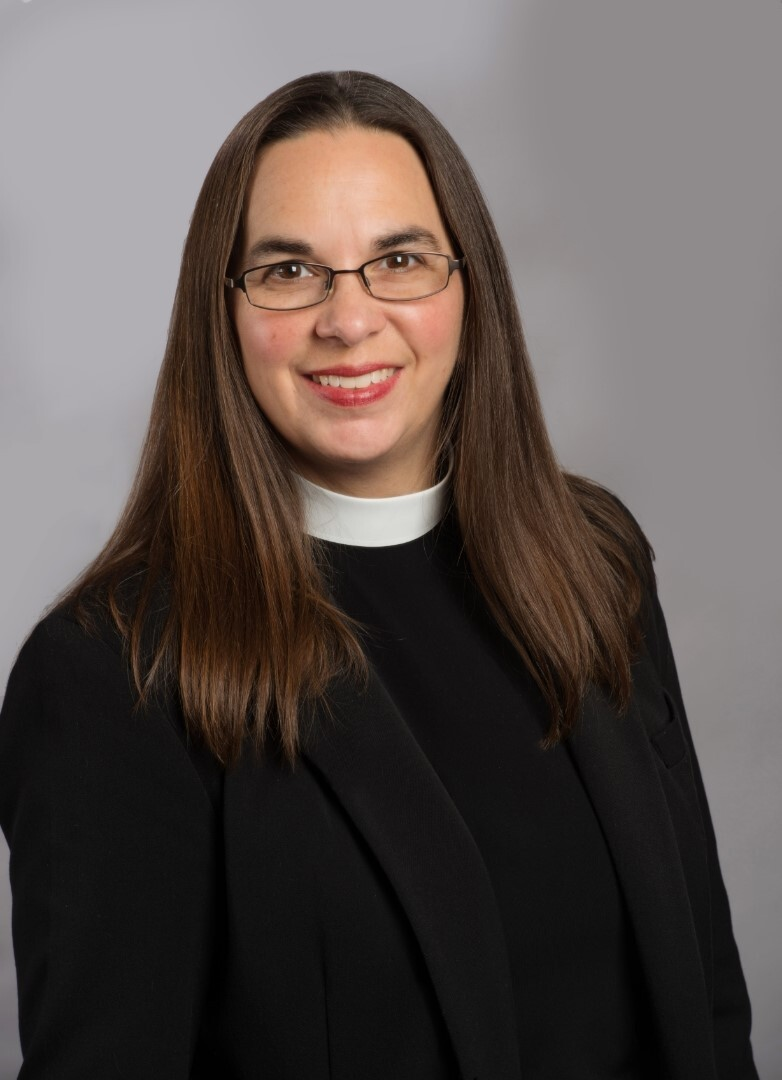St. Mark Calls New Associate Pastor
On Sunday, June 6th, St. Mark members voted resoundingly to extend a call to Katrina Steingraber to be our new associate pastor! I am so thankful to our call committee for the work they put into this important decision, and to everyone who took the time to either vote early or attend the meeting, which was held in our new outdoor worship space on the lawn. We began this pastoral transition process over a year ago, and Katrina’s presence in our congregation will be the final step of the journey. Please join me in welcoming Katrina as she begins her ministry at St. Mark on Sunday, July 4th!
Peace, Rev. Chad McKenna, senior pastor

 table div table+table+table+table+table+table+table+table+table div table{width:100%;padding:0}table div table+table+table+table+table+table+table+table+table div table img{width:96.23%;padding:0;float:none}table div table+table+table+table+table+table+table+table+table div table td{width:100%;padding:0 1.88% 18px}/* styles */Join us for Worship Sunday - 3rd Sunday after Pentecost & Confirmation Sunday
9:00 am - Traditional Worship in the Sanctuary
11:00 am - Blended Worship on the Lawn- Bring your lawn chair or blanket, or you can stay in your car.

Our weekly live-stream will occur during the 9:00 am Sanctuary service.

As of May 30th, you no longer need to register for the 9:00 am service. Though we encourage you to register in advance for the sake of our Sunday volunteers and staff, you also have the option of simply coming to worship on Sunday morning. Ushers will continue to take temperatures and sign in all our worshippers until Illinois has entered Phase 5.

Please enter through the west doors (Sanctuary doors) for this service.

Follow this link for the live-stream service Sunday.
While the 9:00 am service will be fully traditional, our 11:00 am service outside will have the same musical feel as all our worship this past year, with a blend of traditional and contemporary music. As always, weather-related changes to our outdoor worship will be announced the evening before.

 table div table+table+table+table+table+table+table+table+table+table+table div table{width:100%;padding:0}table div table+table+table+table+table+table+table+table+table+table+table div table img{width:96.23%;padding:0;float:none}table div table+table+table+table+table+table+table+table+table+table+table div table td{width:100%;padding:0 1.88% 18px}/* styles */Confirmation Sunday
We will continue this Sunday with affirming the faith of our 2020 Confirmation class, at the 9:00 worship service. Due to the pandemic, we were not able to have Affirmation Sunday last October. Join us as they take their next step in their faith journey.

 table div table+table+table+table+table+table+table+table+table+table+table+table+table div table{width:100%;padding:0}table div table+table+table+table+table+table+table+table+table+table+table+table+table div table img{width:96.23%;padding:0;float:none}table div table+table+table+table+table+table+table+table+table+table+table+table+table div table td{width:100%;padding:0 1.88% 18px}/* styles */Worship on the Lawn
Join us on the lawn by the playground every Sunday at 11:00 for worship. Grab your lawn chair, blanket, and your family as we worship together. Let's get outside to enjoy St. Mark's beautiful outdoors.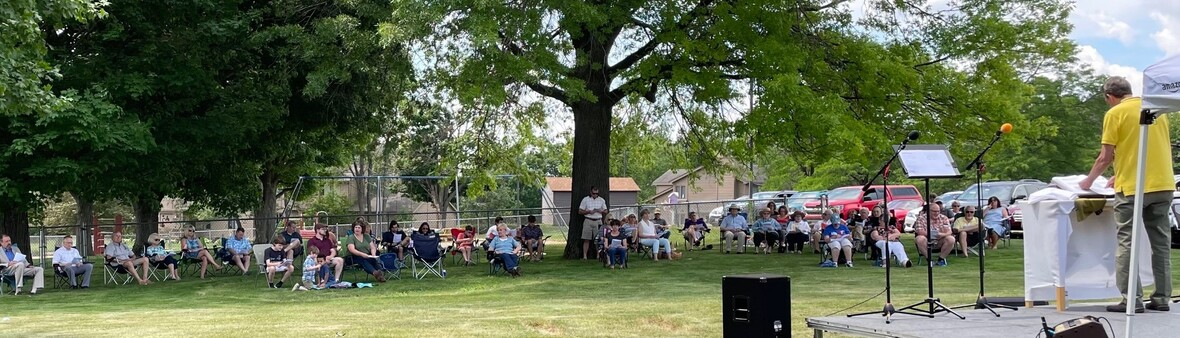table div table+table+table+table+table+table+table+table+table+table+table+table+table+table+table+table div table{width:100%;padding:0}table div table+table+table+table+table+table+table+table+table+table+table+table+table+table+table+table div table img{width:96.23%;padding:0;float:none}table div table+table+table+table+table+table+table+table+table+table+table+table+table+table+table+table div table td{width:100%;padding:0 1.88% 18px}/* styles */Coffee and Donuts are Back!
Our fellowship hour will be between our two worship services from 10:00 - 11:00 a.m. outside under the canopy at the south doors. Libby Malenchik is our hospitality host and will be ready to see you Sunday. Come for a donut, coffee and conversation outside or you may find a table in the cafe.

 table div table+table+table+table+table+table+table+table+table+table+table+table+table+table+table+table+table+table div table{width:100%;padding:0}table div table+table+table+table+table+table+table+table+table+table+table+table+table+table+table+table+table+table div table img{width:96.23%;padding:0;float:none}table div table+table+table+table+table+table+table+table+table+table+table+table+table+table+table+table+table+table div table td{width:100%;padding:0 1.88% 18px}/* styles */St. Mark Mobile App!
Download our free mobile app on the app store or google play. If you are having trouble downloading or have any questions, please call Jill Davenport at 815-218-3403 or email jill@stmarklc.com, she will help you through the process. This is different than our church directory app. We encourage you to download the app. It's about getting involved and staying connected.

Click on this link for information on how to download the app!

 table div table+table+table+table+table+table+table+table+table+table+table+table+table+table+table+table+table+table+table+table div table{width:100%;padding:0}table div table+table+table+table+table+table+table+table+table+table+table+table+table+table+table+table+table+table+table+table div table img{width:96.23%;padding:0;float:none}table div table+table+table+table+table+table+table+table+table+table+table+table+table+table+table+table+table+table+table+table div table td{width:100%;padding:0 1.88% 18px}/* styles */VBS Preview!
Please join us outside between services on June 27 for a preview of Discovery on Adventure Island, our upcoming Vacation Bible School program. We’ll have music and fun out on the lawn, plus frozen treats for everyone!

Register now at MyVBS.org/StMarkLC or at the event. Cost is just \$20 per child (\$50 max/family), and scholarships are available – contact julie@stmarklc.com for details.

 table div table+table+table+table+table+table+table+table+table+table+table+table+table+table+table+table+table+table+table+table+table+table div table{width:100%;padding:0}table div table+table+table+table+table+table+table+table+table+table+table+table+table+table+table+table+table+table+table+table+table+table div table img{width:96.23%;padding:0;float:none}table div table+table+table+table+table+table+table+table+table+table+table+table+table+table+table+table+table+table+table+table+table+table div table td{width:100%;padding:0 1.88% 18px}/* styles *//* styles */ We need YOU to sign up and say you can help on Saturday, June 26. Please call the church office to volunteer at 815-398-3557.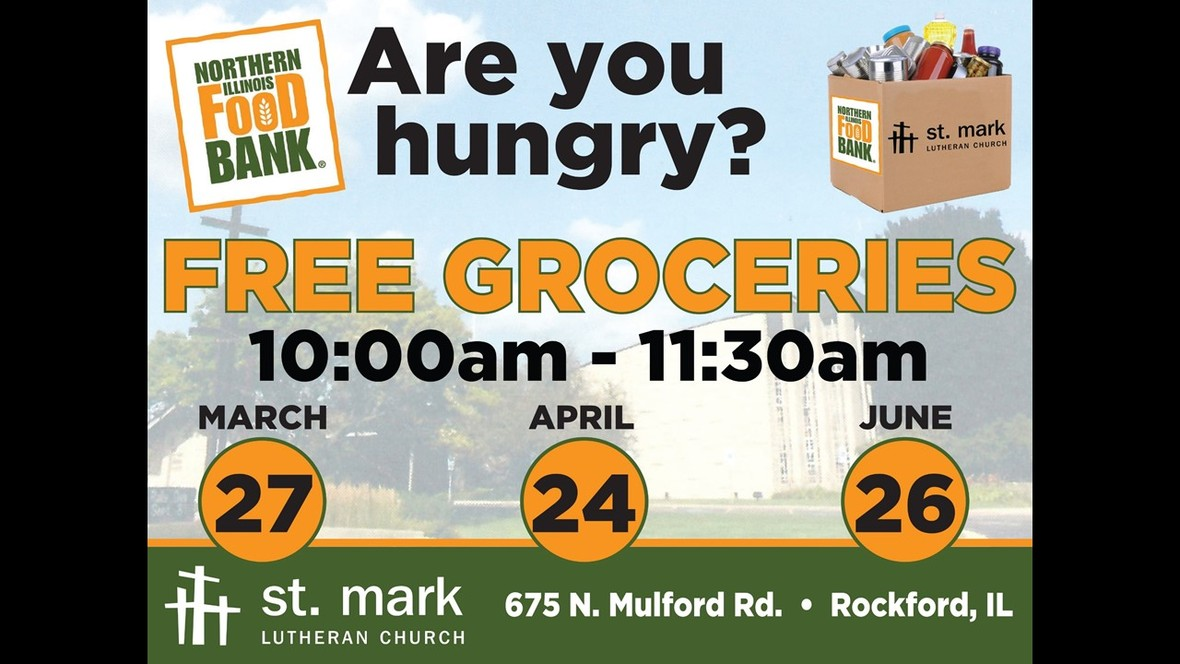table div table+table+table+table+table+table+table+table+table+table+table+table+table+table+table+table+table+table+table+table+table+table+table+table+table div table{width:100%;padding:0}table div table+table+table+table+table+table+table+table+table+table+table+table+table+table+table+table+table+table+table+table+table+table+table+table+table div table img{width:96.23%;padding:0;float:none}table div table+table+table+table+table+table+table+table+table+table+table+table+table+table+table+table+table+table+table+table+table+table+table+table+table div table td{width:100%;padding:0 1.88% 18px}/* styles */Sign up for Altar Flowers

There are some dates still available to sponsor a flower arrangement on Sundays. This can be in honor or memory of someone or just because. The price for one bouquet is \$35.

To sign up for flowers you can call the office at 815-398-3557, or click on this link. If you sign up online, put your memory or honor of in the comment line and pay here or write a check to St. Mark.

If you have the mobile app you can do all of it on there.
You may take your flowers home after the service or stop by the office during the week to pick them up.

 table div table+table+table+table+table+table+table+table+table+table+table+table+table+table+table+table+table+table+table+table+table+table+table+table+table+table+table div table{width:100%;padding:0}table div table+table+table+table+table+table+table+table+table+table+table+table+table+table+table+table+table+table+table+table+table+table+table+table+table+table+table div table img{width:96.23%;padding:0;float:none}table div table+table+table+table+table+table+table+table+table+table+table+table+table+table+table+table+table+table+table+table+table+table+table+table+table+table+table div table td{width:100%;padding:0 1.88% 18px}/* styles */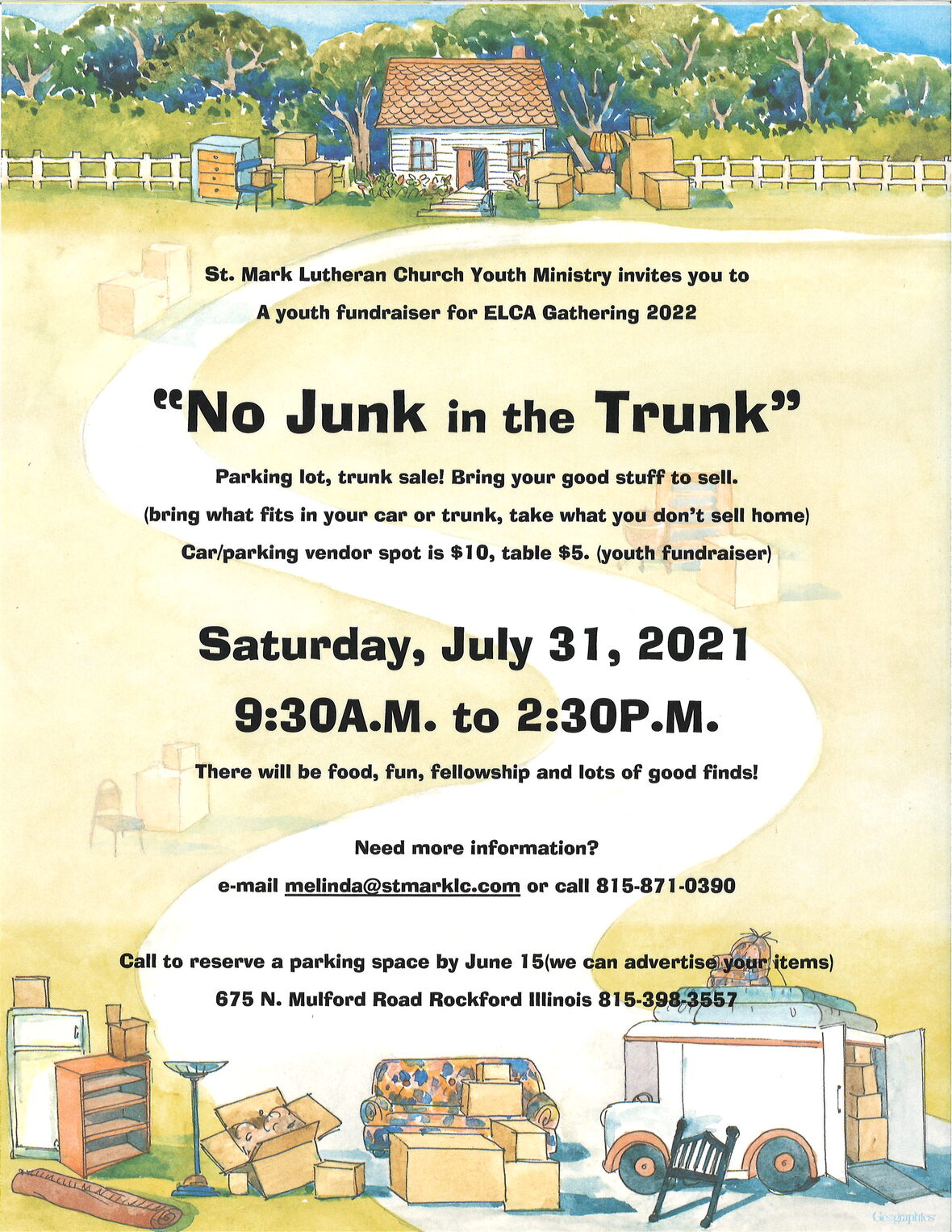“One man’s trash is another man’s treasure.”
People are signing up for our parking lot sale. Have you considered signing up? This week we have people that have said they would have antiques, another will have young children’s clothes, and we even have a new room air conditioner. Sign-up, bring your stuff, and a chair to sit on. We will have lunch available to purchase.

 table div table+table+table+table+table+table+table+table+table+table+table+table+table+table+table+table+table+table+table+table+table+table+table+table+table+table+table+table+table+table div table{width:100%;padding:0}table div table+table+table+table+table+table+table+table+table+table+table+table+table+table+table+table+table+table+table+table+table+table+table+table+table+table+table+table+table+table div table img{width:96.23%;padding:0;float:none}table div table+table+table+table+table+table+table+table+table+table+table+table+table+table+table+table+table+table+table+table+table+table+table+table+table+table+table+table+table+table div table td{width:100%;padding:0 1.88% 18px}/* styles */Katie’s Cup – coffee, conversation and community at 502 7th Street

Thursdays 9:00 - 1:00 - Midtown Farmers Market
Thursdays 9:15 Walking Club
June 10 – 10 am Book Club “The Spellman Files’ by Lisa Lutz.
June 26 - 8:00 - 11:00 - Bike the City with Gary Anderson
July 3 - 9:00 - noon - Cars and Coffee

 table div table+table+table+table+table+table+table+table+table+table+table+table+table+table+table+table+table+table+table+table+table+table+table+table+table+table+table+table+table+table+table+table div table{width:100%;padding:0}table div table+table+table+table+table+table+table+table+table+table+table+table+table+table+table+table+table+table+table+table+table+table+table+table+table+table+table+table+table+table+table+table div table img{width:96.23%;padding:0;float:none}table div table+table+table+table+table+table+table+table+table+table+table+table+table+table+table+table+table+table+table+table+table+table+table+table+table+table+table+table+table+table+table+table div table td{width:100%;padding:0 1.88% 18px}/* styles */Thank you to our members for their steady giving to St. Mark in 2021! It has been a blessed year for St. Mark so far. Envelope offerings have exceeded our budget projections through May despite the challenges of worship during the pandemic. This year, we will be sending out mid-year giving statements for you to compare with your records. The statements will be sent by e-mail or in the mail to those members without e-mail addresses. If you would prefer to receive your statement in the mail, please notify the church office at 815-398-3557.

 table div table+table+table+table+table+table+table+table+table+table+table+table+table+table+table+table+table+table+table+table+table+table+table+table+table+table+table+table+table+table+table+table+table+table div table{width:100%;padding:0}table div table+table+table+table+table+table+table+table+table+table+table+table+table+table+table+table+table+table+table+table+table+table+table+table+table+table+table+table+table+table+table+table+table+table div table img{width:96.23%;padding:0;float:none}table div table+table+table+table+table+table+table+table+table+table+table+table+table+table+table+table+table+table+table+table+table+table+table+table+table+table+table+table+table+table+table+table+table+table div table td{width:100%;padding:0 1.88% 18px}/* styles */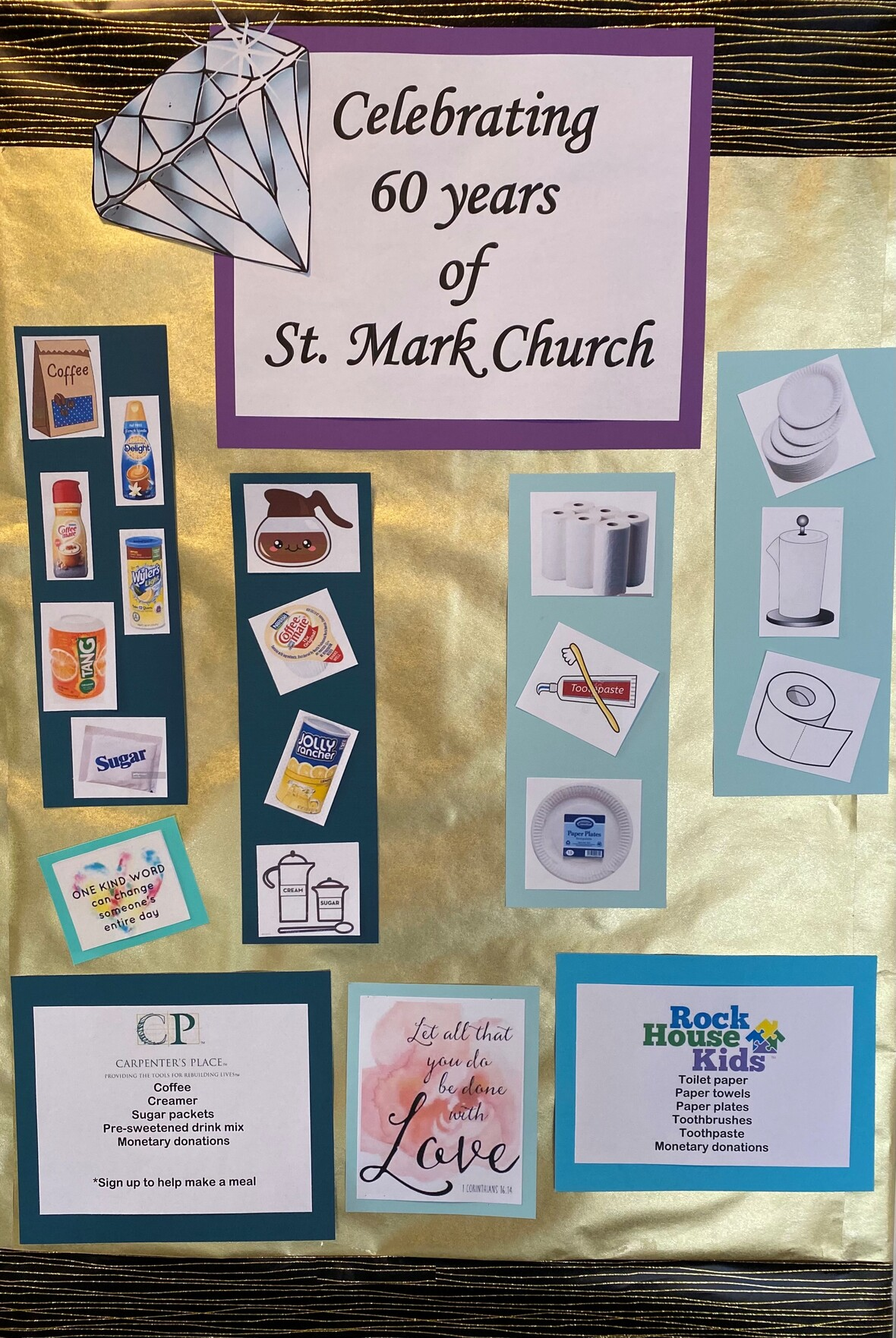table div table+table+table+table+table+table+table+table+table+table+table+table+table+table+table+table+table+table+table+table+table+table+table+table+table+table+table+table+table+table+table+table+table+table+table+table div table{width:100%;padding:0}table div table+table+table+table+table+table+table+table+table+table+table+table+table+table+table+table+table+table+table+table+table+table+table+table+table+table+table+table+table+table+table+table+table+table+table+table div table img{width:96.23%;padding:0;float:none}table div table+table+table+table+table+table+table+table+table+table+table+table+table+table+table+table+table+table+table+table+table+table+table+table+table+table+table+table+table+table+table+table+table+table+table+table div table td{width:100%;padding:0 1.88% 18px}/* styles *//* styles */ Celebrating 60 years of St. Mark Church All during the month of June, we are collecting the items listed below for Rock House Kids and Carpenter’s Place. There is a bin by the canopy doors or the “red” truck in Fellowship Hall. Carpenter’s Place: coffee, creamer, sugar packets, pre-sweetened drink mixes. Rock House Kids: toilet paper, paper towels, paper plates, toothbrushes, toothpaste. Monetary donations are also welcome. You can donate on the church giving app, or give here. St. Mark delivers meals to Carpenter’s Place six times a year. Carpenter’s Place help those suffering from homelessness find peace and hope. St. Mark members make and deliver food to Rock House Kids once a month. Rock House Kids is feeds at-risk children physically, spiritually and emotionally. Read more about Rockhousekids.org and Carpentersplace.org
 table div table+table+table+table+table+table+table+table+table+table+table+table+table+table+table+table+table+table+table+table+table+table+table+table+table+table+table+table+table+table+table+table+table+table+table+table+table+table div table{width:100%;padding:0}table div table+table+table+table+table+table+table+table+table+table+table+table+table+table+table+table+table+table+table+table+table+table+table+table+table+table+table+table+table+table+table+table+table+table+table+table+table+table div table img{width:96.23%;padding:0;float:none}table div table+table+table+table+table+table+table+table+table+table+table+table+table+table+table+table+table+table+table+table+table+table+table+table+table+table+table+table+table+table+table+table+table+table+table+table+table+table div table td{width:100%;padding:0 1.88% 18px}/* styles */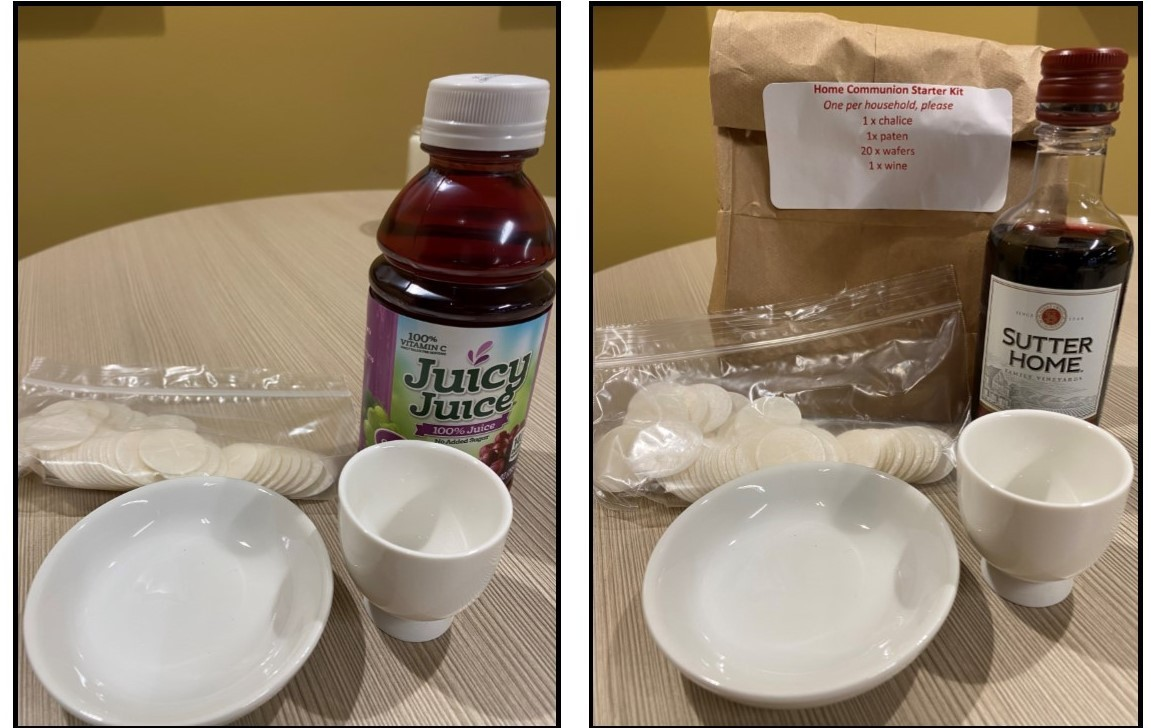Home Communion Kits
If you need to replenish your communion supplies, please stop by the church during office hours, Monday - Friday 9:00 am - 4:00 pm. If you need to come by after these hours, please call the church office at 815-398-3557. Watch this short video from Pastor Chad or read more here.

 table div table+table+table+table+table+table+table+table+table+table+table+table+table+table+table+table+table+table+table+table+table+table+table+table+table+table+table+table+table+table+table+table+table+table+table+table+table+table+table+table div table{width:100%;padding:0}table div table+table+table+table+table+table+table+table+table+table+table+table+table+table+table+table+table+table+table+table+table+table+table+table+table+table+table+table+table+table+table+table+table+table+table+table+table+table+table+table div table img{width:96.23%;padding:0;float:none}table div table+table+table+table+table+table+table+table+table+table+table+table+table+table+table+table+table+table+table+table+table+table+table+table+table+table+table+table+table+table+table+table+table+table+table+table+table+table+table+table div table td{width:100%;padding:0 1.88% 18px}/* styles */St. Mark Prayer Chain
Would you like to be a part of the St. Mark prayer chain? Prayer requests come into the church office weekly. As we receive prayer requests we would send out an e-mail and ask that you pray daily for the persons mentioned in the requests. All requests are to remain confidential. Please e-mail melinda@stmarklc.com if you would like to participate in the prayer chain. “Be anxious for nothing, but in everything by prayer and supplication, with thanksgiving, let your requests be made known to God.” Colossians 4:2.

 table div table+table+table+table+table+table+table+table+table+table+table+table+table+table+table+table+table+table+table+table+table+table+table+table+table+table+table+table+table+table+table+table+table+table+table+table+table+table+table+table+table+table div table{width:100%;padding:0}table div table+table+table+table+table+table+table+table+table+table+table+table+table+table+table+table+table+table+table+table+table+table+table+table+table+table+table+table+table+table+table+table+table+table+table+table+table+table+table+table+table+table div table img{width:96.23%;padding:0;float:none}table div table+table+table+table+table+table+table+table+table+table+table+table+table+table+table+table+table+table+table+table+table+table+table+table+table+table+table+table+table+table+table+table+table+table+table+table+table+table+table+table+table+table div table td{width:100%;padding:0 1.88% 18px}/* styles */## Youth MinistryHigh School Youth and those entering high school in the fall are invited on our Kindness Matters Tour.
Come learn about outreach in our congregation, to our neighbors, and in the community. We will be out in the community each day. Please sign-up by Sunday, June 13. (we need to know t-shirt size) You can message Melinda Alekna at 815-871-0390. Cost is \$50 and maybe paid on the St. Mark app under giving, or may be paid in the church office.

Parents and Youth who are entering 8th grade through high school…
Boundless…God Beyond Measure. ELCA Youth Gathering 2022 registration happens in September 2021. Please join us Sunday, June 27 at noon near the church playground. (bring a chair) We will have information sharing and time for questions. Our youth typically have one opportunity to attend the Gathering while in high school. Please e-mail melinda@stmarklc.comor call 815-871-0390.

 table div table+table+table+table+table+table+table+table+table+table+table+table+table+table+table+table+table+table+table+table+table+table+table+table+table+table+table+table+table+table+table+table+table+table+table+table+table+table+table+table+table+table+table+table+table div table{width:100%;padding:0}table div table+table+table+table+table+table+table+table+table+table+table+table+table+table+table+table+table+table+table+table+table+table+table+table+table+table+table+table+table+table+table+table+table+table+table+table+table+table+table+table+table+table+table+table+table div table img{width:96.23%;padding:0;float:none}table div table+table+table+table+table+table+table+table+table+table+table+table+table+table+table+table+table+table+table+table+table+table+table+table+table+table+table+table+table+table+table+table+table+table+table+table+table+table+table+table+table+table+table+table+table div table td{width:100%;padding:0 1.88% 18px}/* styles */## Summer Intern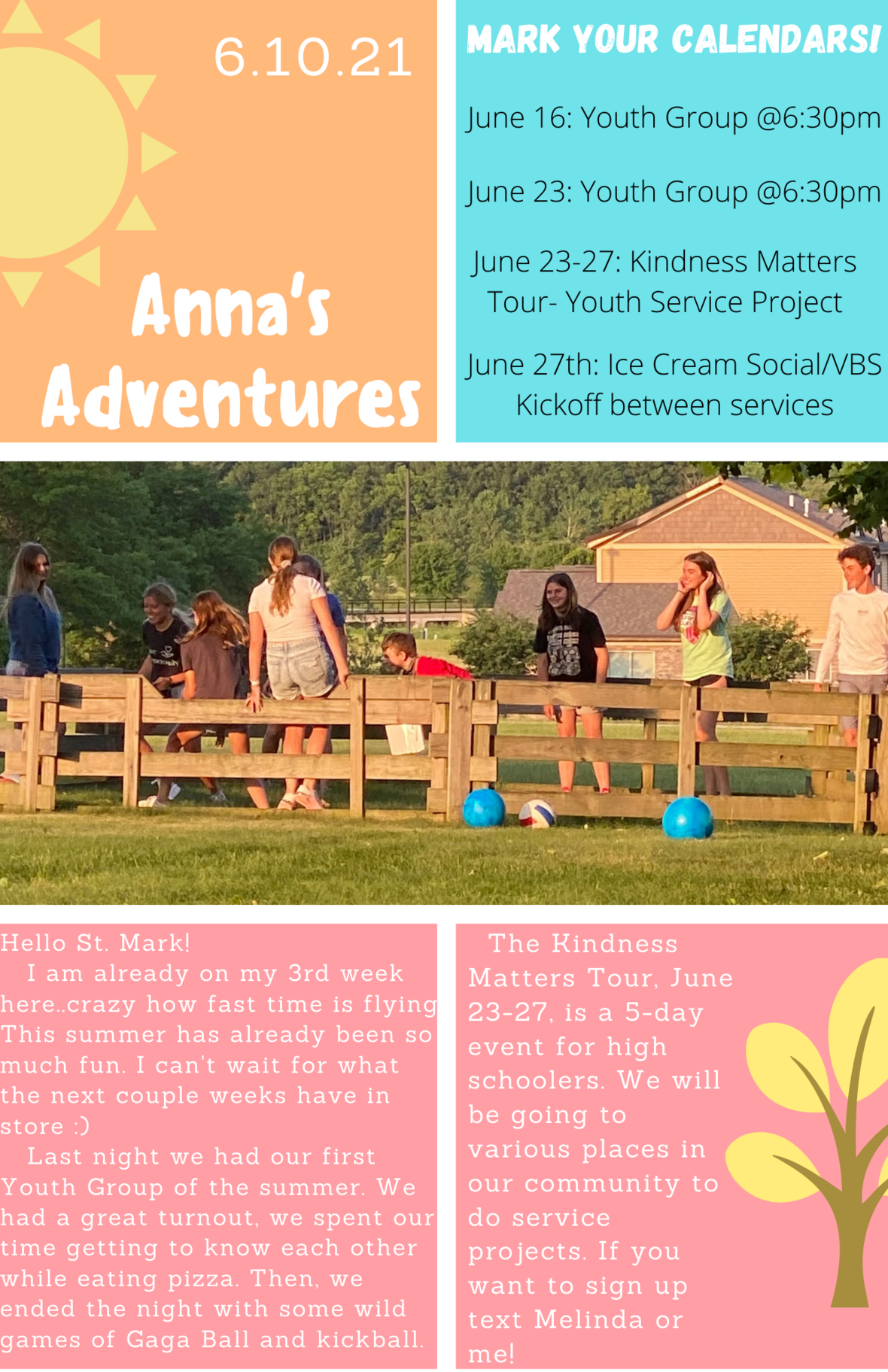table div table+table+table+table+table+table+table+table+table+table+table+table+table+table+table+table+table+table+table+table+table+table+table+table+table+table+table+table+table+table+table+table+table+table+table+table+table+table+table+table+table+table+table+table+table+table+table+table div table{width:100%;padding:0}table div table+table+table+table+table+table+table+table+table+table+table+table+table+table+table+table+table+table+table+table+table+table+table+table+table+table+table+table+table+table+table+table+table+table+table+table+table+table+table+table+table+table+table+table+table+table+table+table div table img{width:96.23%;padding:0;float:none}table div table+table+table+table+table+table+table+table+table+table+table+table+table+table+table+table+table+table+table+table+table+table+table+table+table+table+table+table+table+table+table+table+table+table+table+table+table+table+table+table+table+table+table+table+table+table+table+table div table td{width:100%;padding:0 1.88% 18px}/* styles *//* styles */ Congratulations to our high school seniors! Each week we will introduce you to a few of our graduates.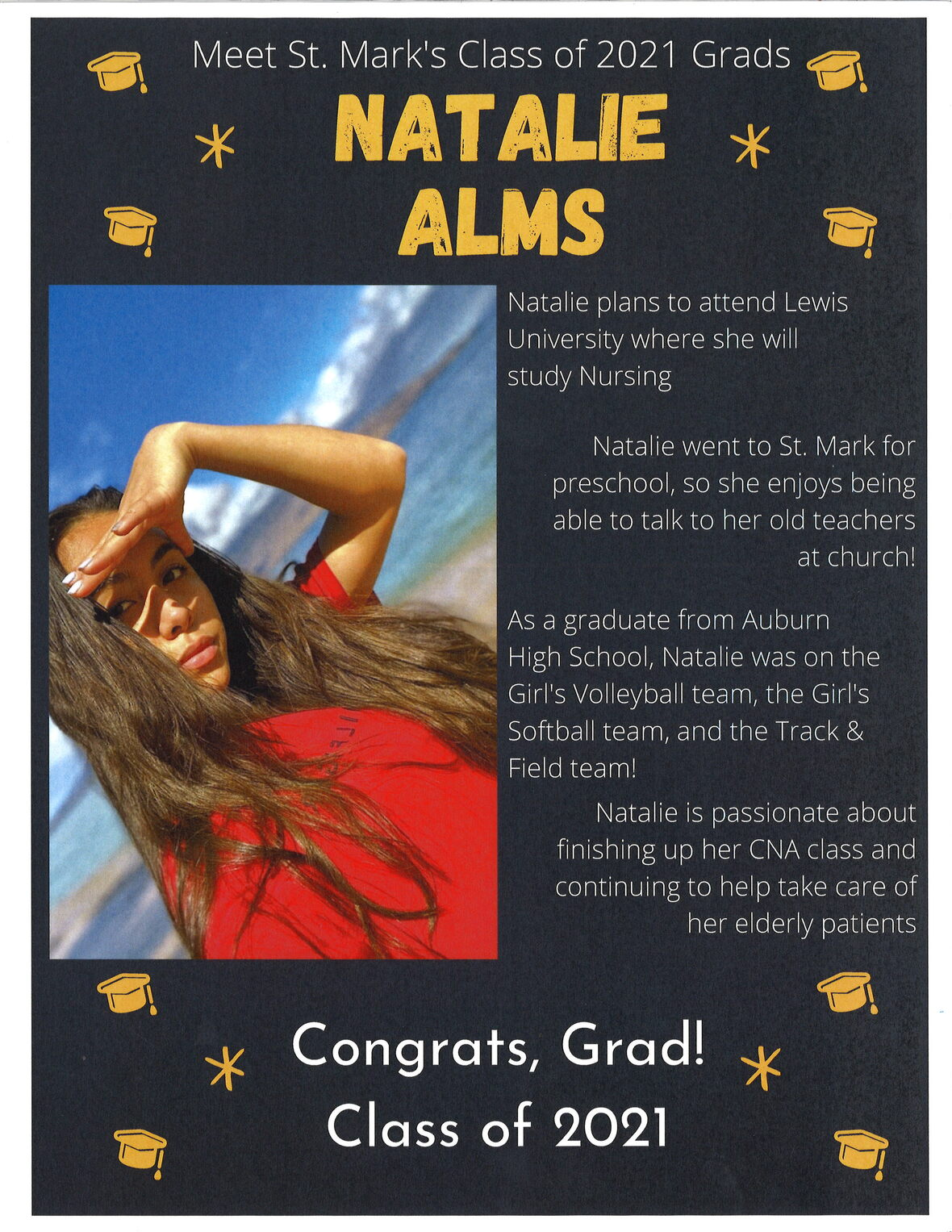table div table+table+table+table+table+table+table+table+table+table+table+table+table+table+table+table+table+table+table+table+table+table+table+table+table+table+table+table+table+table+table+table+table+table+table+table+table+table+table+table+table+table+table+table+table+table+table+table+table+table+table+table div table{width:100%;padding:0}table div table+table+table+table+table+table+table+table+table+table+table+table+table+table+table+table+table+table+table+table+table+table+table+table+table+table+table+table+table+table+table+table+table+table+table+table+table+table+table+table+table+table+table+table+table+table+table+table+table+table+table+table div table img{width:96.23%;padding:0;float:none}table div table+table+table+table+table+table+table+table+table+table+table+table+table+table+table+table+table+table+table+table+table+table+table+table+table+table+table+table+table+table+table+table+table+table+table+table+table+table+table+table+table+table+table+table+table+table+table+table+table+table+table+table div table td{width:100%;padding:0 1.88% 18px}/* styles */## Our St. Mark members:

Tim Panjkovich, Jack Sowl, Jan Stringer, Adleigh Siemans,
Lorine Kortus, Linda Crooks, Jan Cotter, Roger Lind, Diane Swanson, Billie Reinhold, Sherri Lindquist, Bill Jennings, Bette Patterson, Keith Haggestad.

## Family and friends of St. Mark members:

Bob Pollock, Ezra Mark Wiegel, Ed Benfield, Conrad Semmelroth, Nici King, Vicki Kalnins, Kate Cuccaro, Debbie Adams, Wanda Adamson, Milane Bagel, Janet Webster, Rae Ann McIntire, Linda Johnson, Eva Love Sherbondy, Norma Comstock, Carolyn King, Ashton Henley.

## Those serving in the military and their families:

Hunter Haggestad, Tristan Davenport, Scot Hornick,
Bailey Panjkovich, Xander Gehrke, Jim Tammen, Brandon Ballenger, Taylor Eversole, Dean Barron.

Strengthen and encourage those in public health services and in the medical profession: care-givers, nurses, attendants, doctors, all who commit themselves to care for the sick and their families.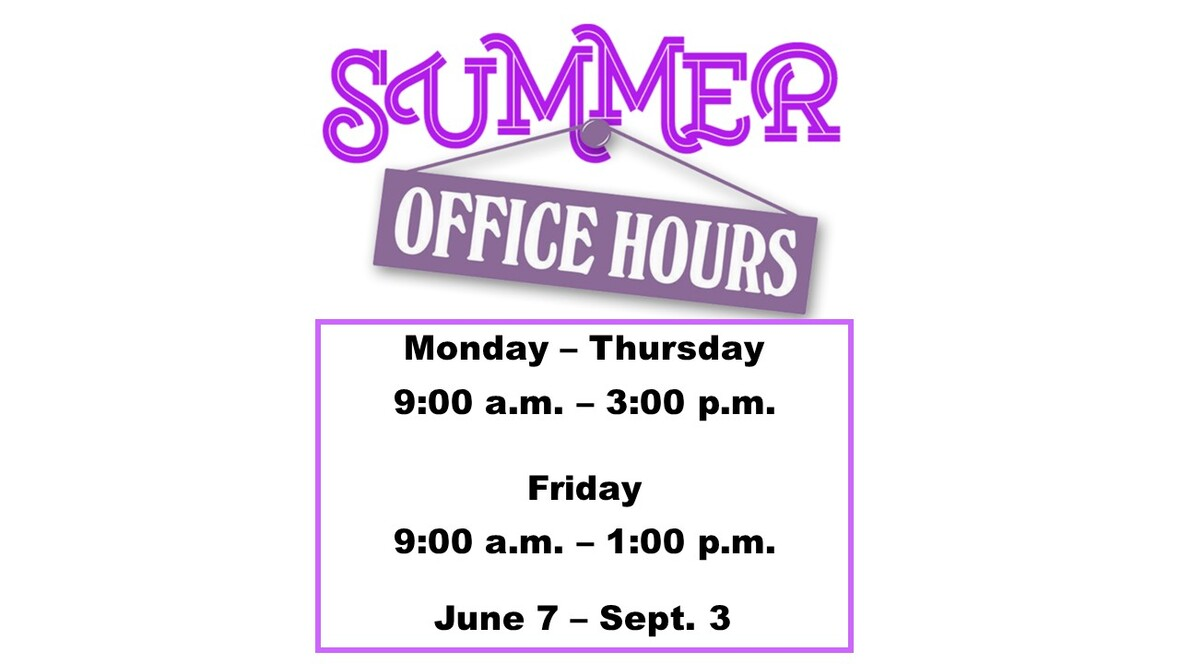table div table+table+table+table+table+table+table+table+table+table+table+table+table+table+table+table+table+table+table+table+table+table+table+table+table+table+table+table+table+table+table+table+table+table+table+table+table+table+table+table+table+table+table+table+table+table+table+table+table+table+table+table+table+table+table+table div table{width:100%;padding:0}table div table+table+table+table+table+table+table+table+table+table+table+table+table+table+table+table+table+table+table+table+table+table+table+table+table+table+table+table+table+table+table+table+table+table+table+table+table+table+table+table+table+table+table+table+table+table+table+table+table+table+table+table+table+table+table+table div table img{width:96.23%;padding:0;float:none}table div table+table+table+table+table+table+table+table+table+table+table+table+table+table+table+table+table+table+table+table+table+table+table+table+table+table+table+table+table+table+table+table+table+table+table+table+table+table+table+table+table+table+table+table+table+table+table+table+table+table+table+table+table+table+table+table div table td{width:100%;padding:0 1.88% 18px}/* styles *//* styles */ Summer Office Hours Monday through Thursday 9:00 A.M. - 3:00 P.M. Friday 9:00 A.M. - 1:00 P.M. Telephone: 815-398-3557 Publications St. Mark Memos, the weekly e-newsletter, is distributed each Thursday. The deadline for submission is Monday by 3:00 P.M. Please click to send submissions. Worship Services Miss a Sunday at St. Mark? Please visit our website and watch the service online. Please click to watch.